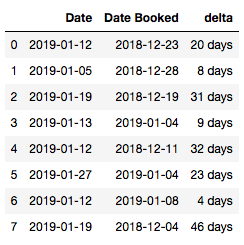# Round Numbers and Days Up in Python

```>> import math
>> math.ceil(1.1)
2

Timedelta('21 days 15:00:00')

>> pd.Timedelta.ceil(df.delta.mean(), freq = 'd')
Timedelta('22 days 00:00:00')```

Use Case:

I have a DataFrame with two sets of dates and the difference between them. There are two different ways to get a rounded up number of days, depending if you want a Timedelta or an integer.Method 1: Integer

```>> df.delta.mean()
Timedelta('21 days 15:00:00')
>> df_ex.delta.mean() / pd.Timedelta(days = 1)
21.625```

That’s the answer, but also for my purposes, partial days is too fine for my purposes. Dividing a Timedelta by pd.Timedelta(days = 1) will result in an integer.

```>> math.ceil(df_ex.delta.mean() / pd.Timedelta(days = 1))
22```

Method 2: Timedelta

```>> df.delta.mean()
Timedelta('21 days 15:00:00')

>> pd.Timedelta.ceil(df.delta.mean(), freq = 'd')
Timedelta('22 days 00:00:00')```